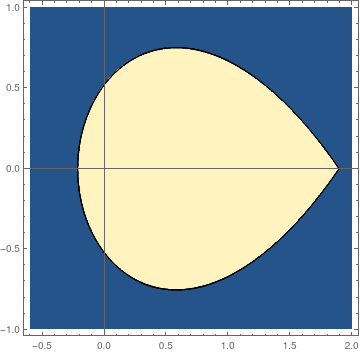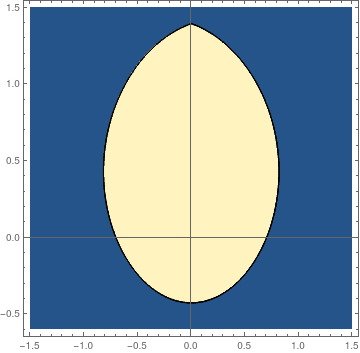# Preface

The influence of a periodic forcing term on the Duffing equation is considered in this section.

Introduction to Linear Algebra with Mathematica

# Forced Duffing equationWe plot the stability boundary for the forced Duffing equation $\ddot{x} + x(t) + \frac{1}{6}\, x^3 = \frac{1}{3}\,\cos \left( \frac{3t}{5} \right) , \qquad x(0) =a, \quad \dot{x}(0) = b .$ pfun = ParametricNDSolveValue[ {x''[t] == -x[t] + x[t]^3/6 + (1/3)*Cos[3*t/5], x == a, x' == b}, x, {t, 0, 100}, {a, b}]; fun[a_?NumericQ, b_?NumericQ] := Module[ {res}, res = Quiet[pfun[a, b]]; Boole[res["Domain"] === {{0., 100.}}] ]; plot = ContourPlot[fun[a, b], {a, -0.6, 2}, {b, -1, 1}, PlotPoints -> 50, MaxRecursion -> 3, Axes -> True, AxesOrigin -> {0, 0}] Stability boundary. Mathematica codes.We plot the stability boundary for the forced Duffing equation $\ddot{x} + x(t) + \frac{1}{6}\, x^3 = \frac{1}{3}\,\sin \left( \frac{3t}{5} \right) , \qquad x(0) =a, \quad \dot{x}(0) = b .$ pfun = ParametricNDSolveValue[ {x''[t] == -x[t] + x[t]^3/6 + (1/3)*Sin[3*t/5], x == a, x' == b}, x, {t, 0, 100}, {a, b}]; fun[a_?NumericQ, b_?NumericQ] := Module[ {res}, res = Quiet[pfun[a, b]]; Boole[res["Domain"] === {{0., 100.}}] ]; plot = ContourPlot[fun[a, b], {a, -1.5, 1.5}, {b, -0.6, 1.5}, PlotPoints -> 50, MaxRecursion -> 3, Axes -> True, AxesOrigin -> {0, 0}] Stability boundary. Mathematica codes.